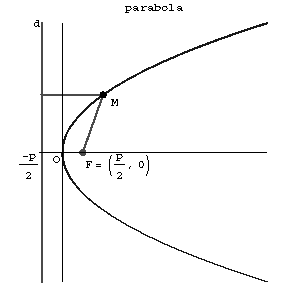# Parabola

A plane curve obtained as the intersection of a circular cone with a plane not passing through the vertex of the cone and parallel to one of its tangent planes. A parabola is a set of points $M$ in the plane for each of which the distance to a given point $F$( the focus of the parabola) is equal to the distance to a certain given line $d$( the directrix). Thus, a parabola is a conic with eccentricity one. The distance $p$ from the focus of the parabola to the directrix is called the parameter. A parabola is a symmetric curve; the point of intersection of a parabola with its axis of symmetry is called the vertex of the parabola, the axis of symmetry is called the axis of the parabola. A diameter of a parabola is any straight line parallel to its axis, and can be defined as the locus of the midpoints of a set of parallel chords.Figure: p071150a

A parabola is a non-central second-order curve. Its canonical equation has the form

$$y ^ {2} = 2px .$$

The equation of the tangent to a parabola at the point $( x _ {0} , y _ {0} )$ is

$$yy _ {0} = p( x + x _ {0} ) .$$

The equation of a parabola in polar coordinates $( \rho , \phi )$ is

$$\rho = \frac{p}{1 - \cos \phi } ,\ \textrm{ where } 0 < \phi < 2 \pi .$$

A parabola has an optical property: Light rays emanating from the focus travel, after reflection in the parabola, parallel to the axis.